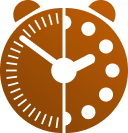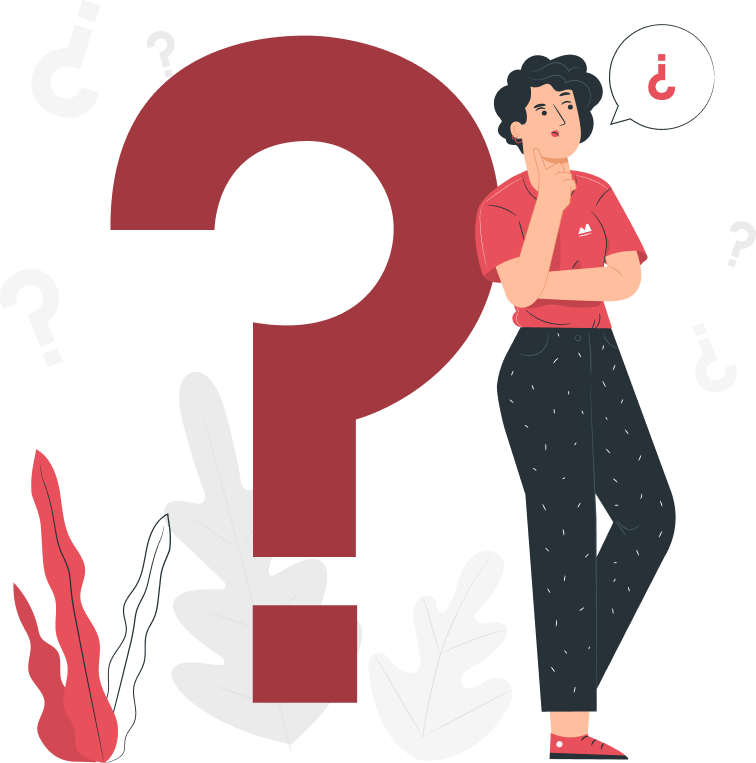# Minutes to Hour - Hours to Minutes

## Convert Minutes to Hours (min to hr) or Hours to Minutes (hr to min)

Home

Time

Min - Hour Converter

You can input in any of the fields and get equivalent values. You can enter time in hours, minutes and sec and convert to hours in decimals
=
:
:

### Formula to convert minutes to hours (mins to hours)

• There are 60 minutes in one hour.
• To convert hours to minutes, you need to multiply by 60.
• #### 1 hour = minutes * 60;

• To convert 2 hours to minutes, you would multiply by 60:

### Formula to convert hours into minutes (hour to min)

• Therefore, to convert minutes to hours, you need to divide by 60.
• #### 1 minute = hour / 60;

• For example, to convert 120 minutes to hours, you would divide by 60:

## Interesting Facts on Hours and Minutes• ### The hour is a unit of time equal to one twenty-fourth of a day.

• The minute and hour are both used in the 12-hour clock and the 24-hour clock.
• The minute and hour are also used in the sexagesimal system of counting, which is based on the number 60.
• The number 60 came about because the ancient Babylonians used a sexagesimal (base 60) counting system. This system was likely developed because 60 is a highly divisible number, with 12 factors (including 1, 2, 3, 4, 5, 6, 10, 12, 15, 20, 30, and 60). This made it ideal for performing complex mathematical calculations.
• The Babylonians used the sexagesimal system to measure time, angles, and other quantities. They divided the day into 12 hours, each of which was divided into 60 minutes. They also divided the circle into 360 degrees, each of which was divided into 60 minutes.
• The Babylonians' sexagesimal system was adopted by the Greeks and Romans, and it is still used today to measure time and angles. For example, we have 60 minutes in an hour and 60 seconds in a minute. We also have 360 degrees in a circle.
• It is not entirely clear why the Babylonians chose the number 60 as the base for their counting system. One possibility is that it was influenced by the fact that they had 12 knuckles on each hand, and that they used their hands to count. Another possibility is that it was influenced by the 12-month lunar calendar.
• The word hour comes from the Latin word hōra, which in turn comes from the Greek word hṓrā (ὥρα). The Greek word hṓrā originally meant "season," but it eventually came to mean "time of day" or "hour."
• The word minute comes from the Latin word pars minuta prima, which means "first small part." This is because a minute is a small part of an hour.
• The words hour and minute entered the English language in the 13th century. The word hour first appeared in Middle English as ure, and the word minute first appeared in Middle English as minute.

## Minute to Hour Converter (min to hr) table## Hours to Minutes Conversion (hr to min) Table## Frequently Asked Questions on Minutes to Hours Calculator